# A Beginner’s Guide to the Bootstrap## Topics

November 22, 2021

What is the bootstrap method?

If you take a quantitative methods course here at Berkeley, chances are that you will learn how to perform a bootstrap. As an introductory data science instructor, it’s one of my favorite topics to teach, not just because it’s a powerful and useful tool, but also because it’s incredibly intuitive. In short, the bootstrap -- also known as resampling with replacement -- allows us to generate a distribution of sample statistics given only a single sample, estimating sampling error.The name of this method is borrowed from the phrase, “pulling yourself up from your bootstraps,” which, when taken literally, is impossible to do. In the same vein, being somehow able to create new samples from a single sample is a seemingly impossible task, but it works! Let’s work through it.

In traditional (i.e. not computational) statistics, we must know the probability distribution of the sample statistic to perform an analysis. These distributions are well-defined and include names you may have heard before: normal, Poisson, and binomial, to name a few. However, inference in a parametric approach usually depends on fulfilling a set of assumptions that may not always be met. Normality, for example, depends on the Central Limit Theorem: the sample must be large and random and the statistic derived from the sample sum or mean.

The issue with this approach arises when we have a statistic that does not meet the assumptions for a known probability distribution. When we don’t know what distribution fits a particular statistic, we are out of luck. In theory, we could go back out in the field and take many more random samples to generate an empirical distribution (i.e. by observation) of the sample statistic, but the issue is that it can take a lot of time, money, and resources to do so.Figure 1. A traditional approach in statistics, outside of using known probability distributions and equations. In this case, we are taking multiple samples of size 60 without replacement (the 3 histograms on the right) directly from the population (the histogram on the left). For each collected sample, we would calculate the sample mean to generate a distribution of sample means.

So, what do we do in that case? We can use the bootstrap, which allows us to move forward even when we don’t know or assume a specific probability distribution. In four short steps, the bootstrap consists of:

1. Taking one large, random sample from the population.

2. Taking another sample with replacement and the same sample size from that original sample (“resampling”).

3. Calculating the statistic of interest from the resample.

4. Repeating steps 2 and 3 many times until we have a distribution of resample statistics.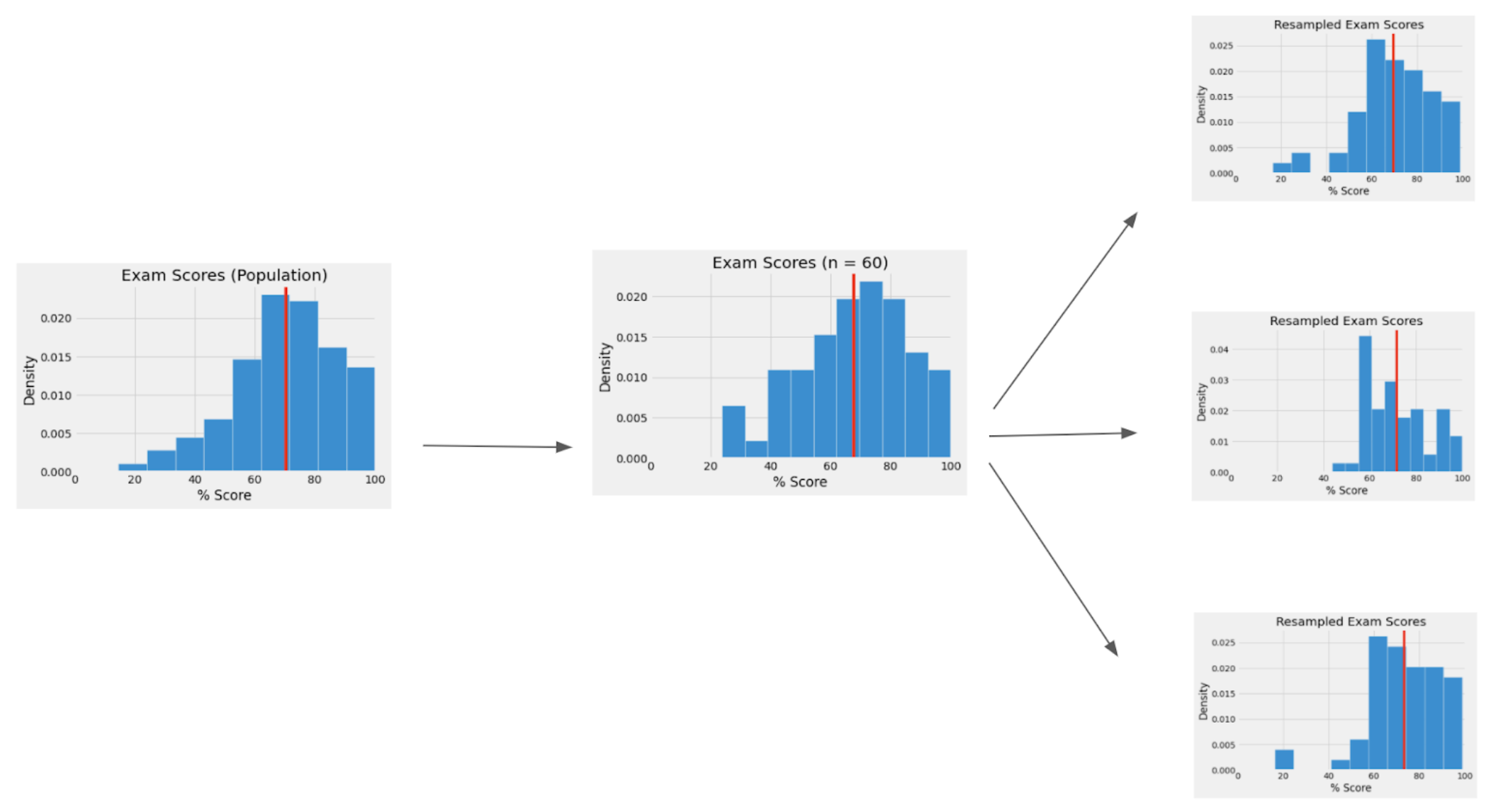Figure 2. The bootstrap approach. Instead of taking multiple samples directly from the population (leftmost histogram), we only have a single, representative sample (the center histogram). We can then generate “new” samples by resampling with replacement from the initial sample (the three rightmost histograms), which allows us to generate the distribution of sample means.

This is incredibly easy given the modern array of computational tools available to us, since performing resamples and calculations of this scale with large datasets is now relatively quick. Once we have this distribution of resample statistics, we can create a confidence interval to estimate the population parameter (which is what I will do in the example below, using Python) or perform a hypothesis test.

Why does it work?

This sounds like magic; how do we create more data from seemingly nowhere? The bootstrap relies on the primary assumption that our initial sample is representative of the population distribution (hence, the sample must be usually large and random). If we do have this representative sample, then the process of resampling with replacement from the initial sample should be roughly equivalent to sampling directly from the population.

In short: “Population → Sample == Sample → Resample” , assuming our sample is representative.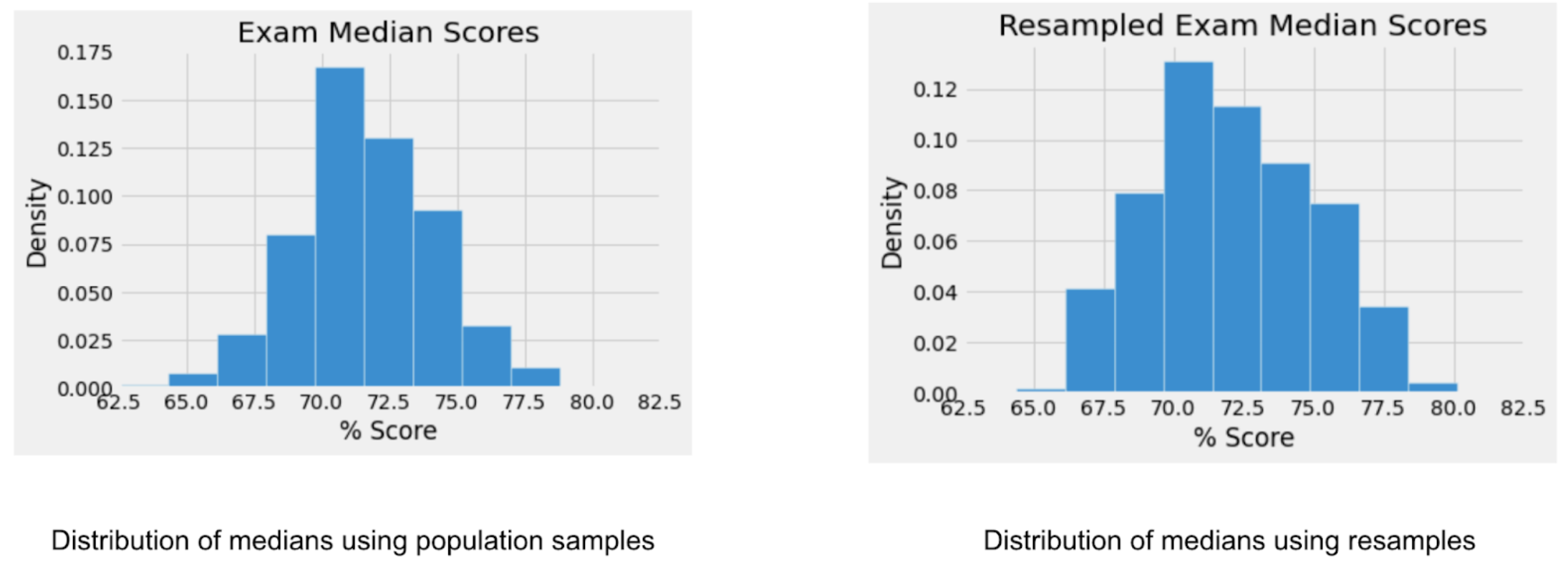Figure 3. Notice the similarities. While they are somewhat different, it shouldn’t matter too much for the final confidence interval. The left graph is generated using the median from 2000 different samples, while the right graph is generated by 2000 different resamples with replacement.

Baking in this sampling error is also the reason why we resample with replacement. When we sample directly from the population, each sample statistic will vary due to random chance and sampling variation. If we resampled without replacement using the exact same sample size, every single resample would be exactly the same; this would only be “shuffling” the data. Using replacement allows us to include this variation in our resamples, as each of our resamples will still look similar to the original sample distribution but not be exactly the same.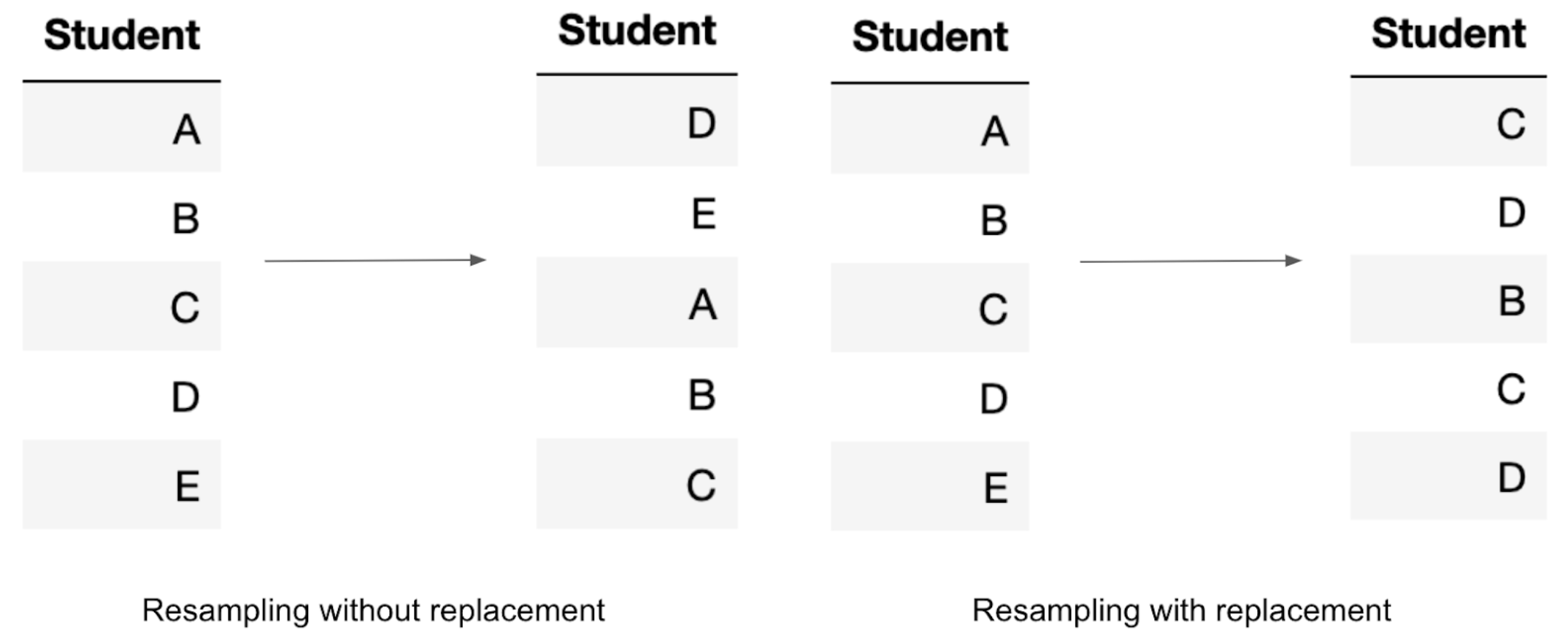Figure 4. In the first case, without replacement, the resample mean would not change. Each time we take a student when resampling without replacement, we remove them from the pool. Therefore, if our resample is the same sample size, all of the students are the same, since everyone is eventually removed from the sampling pool, but are placed in a different order. In the second case, when we resample with replacement, the resampled mean would be slightly different. The same student can be selected multiple times as they are not removed from the pool after selection, so we can end up with a scenario where we exclude Students A and E and repeat students C and D.

An example: Estimating the City of Oakland government’s total salary and benefits

Before we begin, here is a summarized version of the steps to implement a bootstrap in code:

For any sample and statistic, you would...

1. Create a storage object (array, list, vector, etc.).

2. Use a loop that iterates n times (n should be large, scaled on the size of your dataset and the computational power available).

1. In each iteration, generate a resample from the original sample, and then calculate the statistic from that resample.

2. Put that resample statistic into storage.

3. Calculate the confidence interval using the storage object.

Now, let’s put that into practice. Imagine we want to calculate the median salary and benefits of full-time employees working for the City of Oakland in 2018. To do so, we take a random sample without replacement of 40 employees from the population.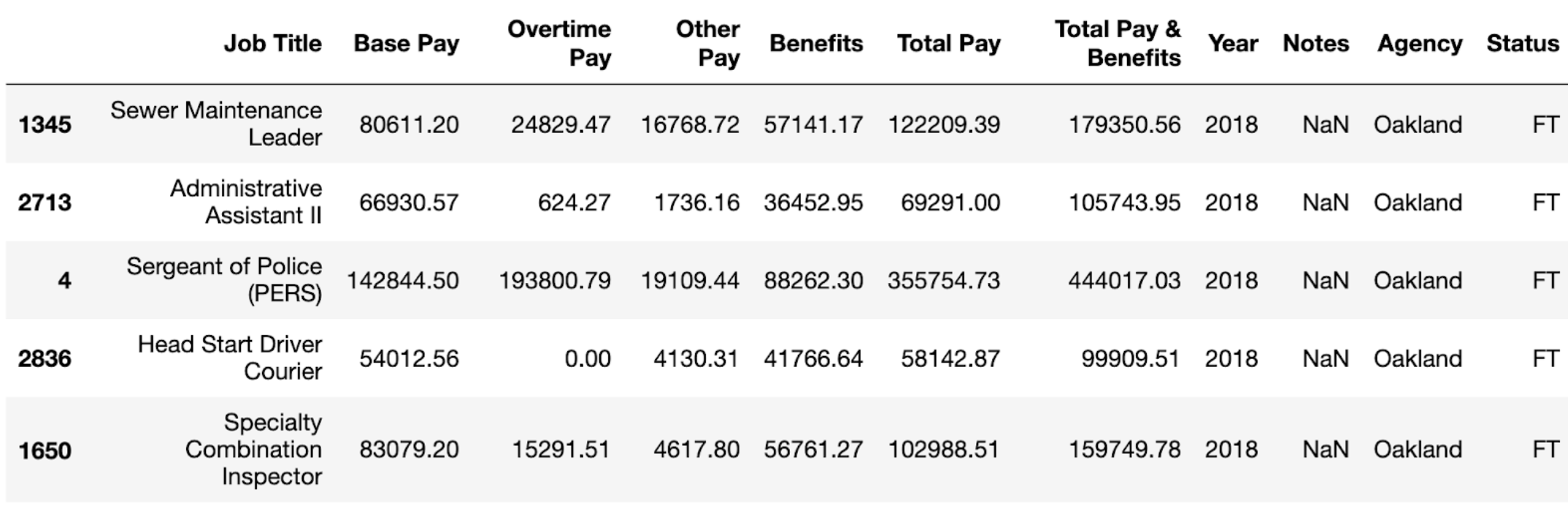The sample distribution is below, and our sample median (yellow line) is \$184,363.25.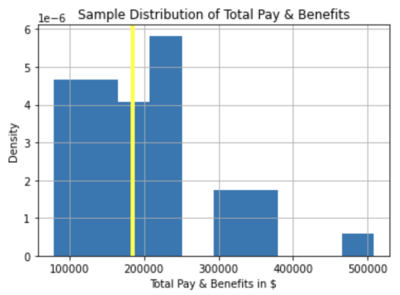As stated previously, we would most likely have received a different sample median and distribution if we took another sample, so we want to account for this by creating a confidence interval to estimate a range of possible population medians for total pay and benefits.

We can do this by following the steps mentioned before: (2) resampling with replacement and the same sample size, (3) calculating the median for each resample, and (4) generating a distribution of medians from the resample. More resamples are better, so in this case, we will use 4000 resamples.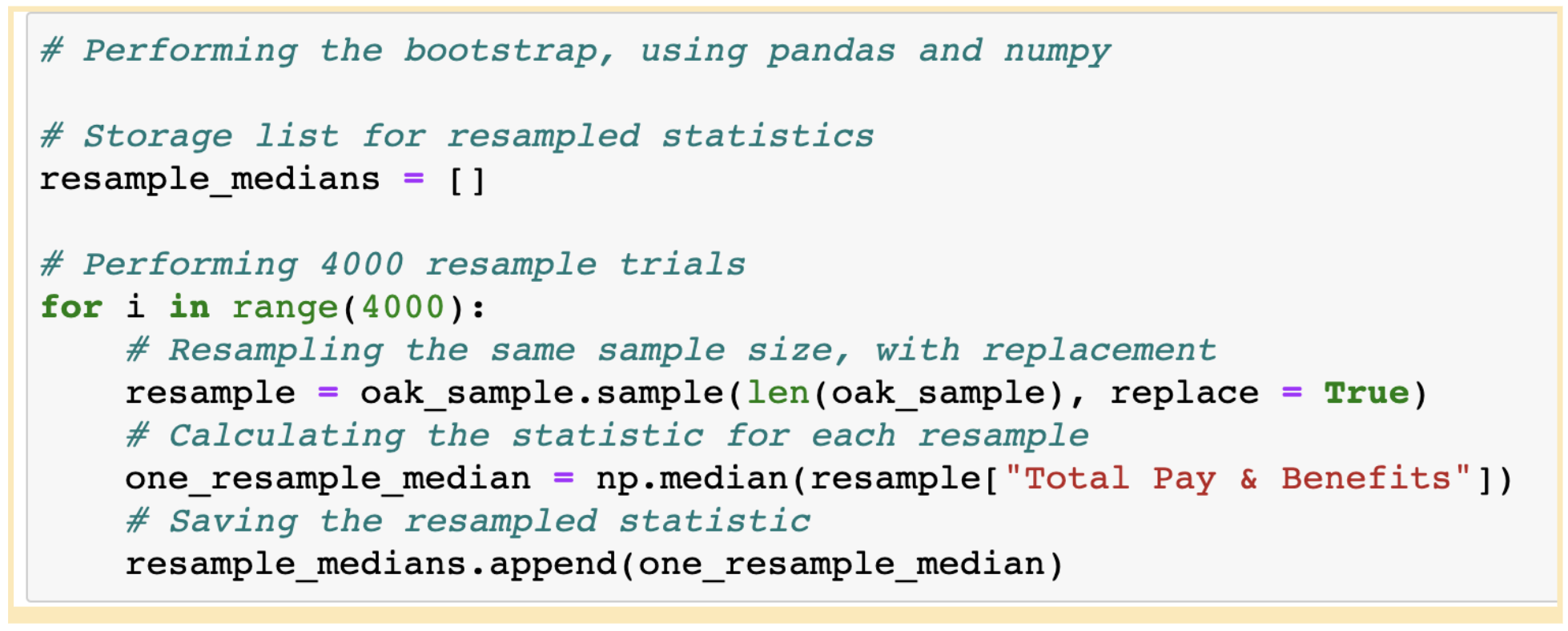Once we have this, we have a distribution of resampled statistics, which allows us to estimate the standard error by simulating sampling variation. We can use this distribution to generate our confidence interval, and in this case, I will use a confidence level of 95%.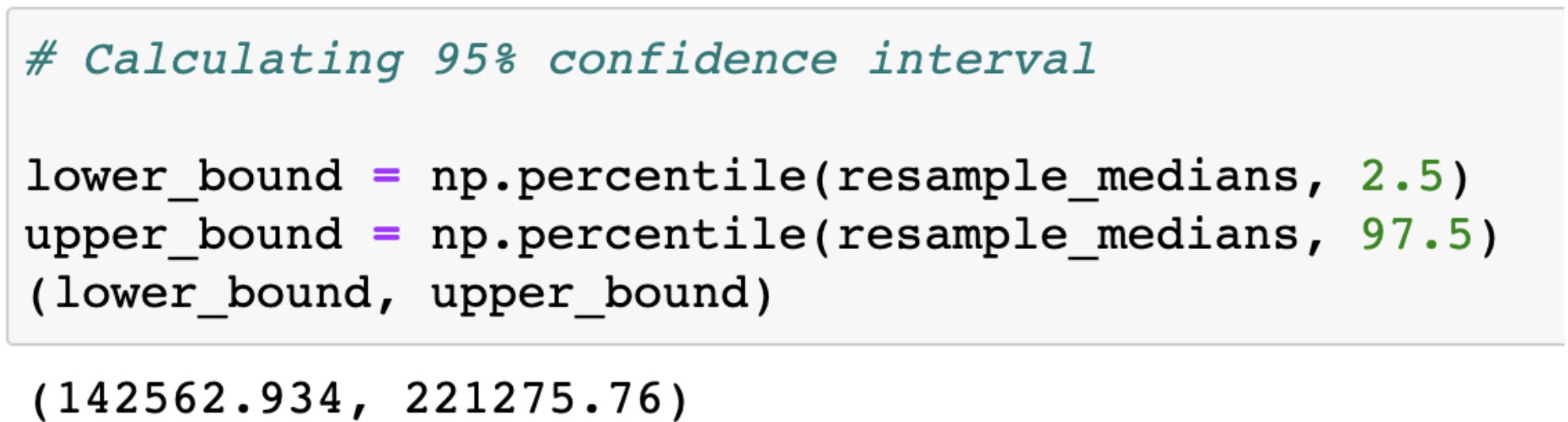Given this information, we would predict that the true population median of total salary and benefits in Oakland is somewhere between \$142,562.93 and \$221,275.76. I’ve included a histogram of this below: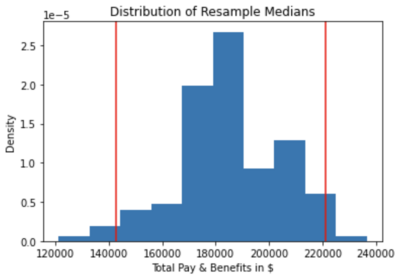For this example, I actually have the full dataset from the City of Oakland’s Open Data Portal, so we do know the true population median and can check if our bootstrap worked correctly! As you can see, the yellow line (the true median) falls between the bounds of the confidence interval, so we did make a correct prediction of the population parameter.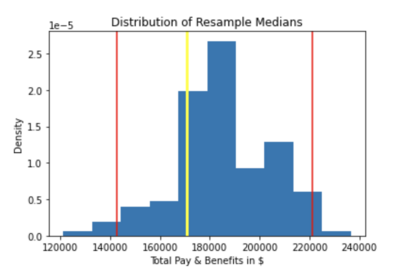Although I did this in Python, it’s easy to do this in any other language, like R. You can also do the bootstrap with a variety of sample types and statistics, such as a list of booleans specifying the correctness of predictions in machine learning and the corresponding prediction accuracy. The steps would be essentially the same.

Acknowledgment

Thank you to Professors John DeNero, Ani Adhikari, and David Wagner for their incredibly useful lesson on bootstrapping in Data 8: Foundations of Data Science. Most of this guide is based on their approach to the topic in that course, which I had the pleasure of working for as a GSI from Fall 2018 to Spring 2021.